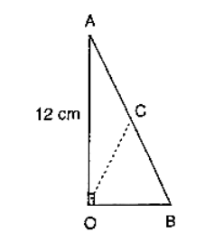# In figure, ∠AOB = 90°, AC = BC, OA = 12 cm and OC = 6.5 cm.

Question:

In figure, ∠AOB = 90°, AC = BC, OA = 12 cm and OC = 6.5 cm. Find the area of ΔAOB.Solution:

Since, the midpoint of the hypotenuse of a right triangle is equidistant from the vertices

∴ CA = CB = OC

⇒ CA = CB = 6.5 cm

⇒ AB = 13 cm

In right angled triangle OAB, we have

$A B^{2}=O B^{2}+O A^{2}$

$\Rightarrow 13^{2}=\mathrm{OB}^{2}+12^{2}$

$\Rightarrow \mathrm{OB}^{2}=13^{2}-12^{2}=169-144=25$

⇒ OB = 5

$\therefore \operatorname{ar}(\triangle \mathrm{AOB})=(1 / 2)(12 \times 5)=30 \mathrm{~cm}^{2}$